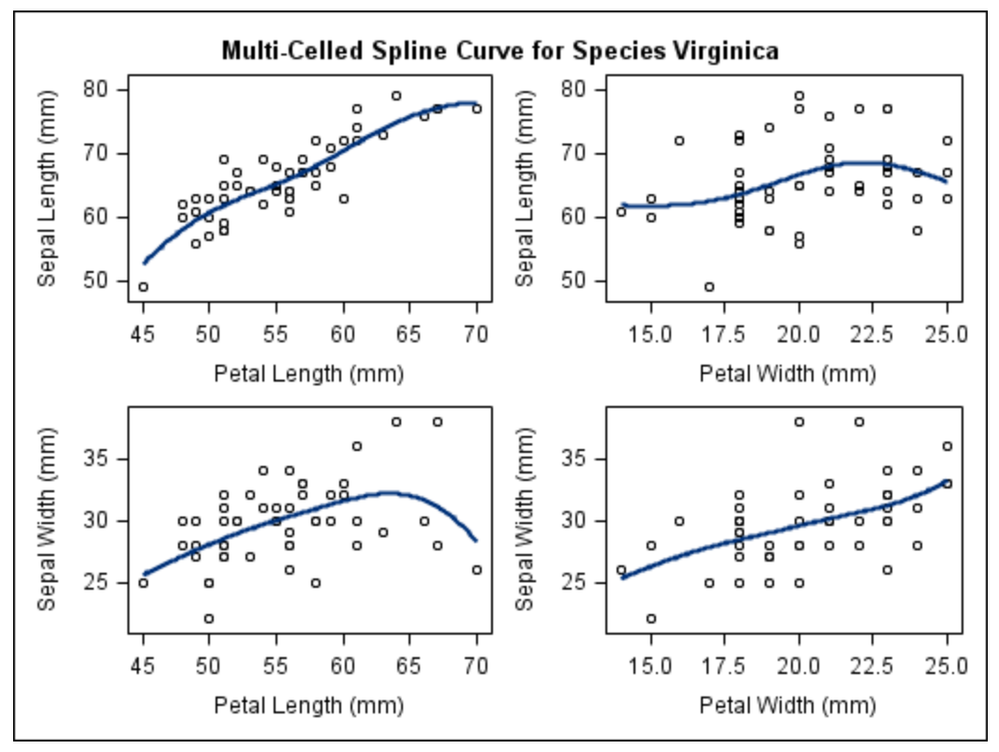## Multiple independent scatter plots

Hi, I'd like to have all 4 of my scatter plots in one window like this:I have 4 scatter plots for 4 different seasons. Here's an example of a scatter plot for winter:

```proc sgplot data=dataname (where=(Month in(12,1,2)));
xaxis label="Temperature (degrees F)";
run;```
1 ACCEPTED SOLUTION

Accepted Solutions

## Re: Multiple independent scatter plots

The image you show is from SGSCATTER online documentation example 2.

Since you want to create a separate graph based on the value of a different variable than is involved in the actual plot then SGPANEL with a custom format for your MONTH variable to get groups probably a good solution. Something like:

```proc format library=work;
value season
12,1,2 = 'Winter'
3,4,5 = 'Spring'
6,7,8 = 'Summer'
9,10,11 = 'Fall';
run;
proc sgpanel data=dataname ;
panelby month /layout=panel rows=2 columns=2 onepanel ;
format month season.;
colaxis label="Temperature (degrees F)";
run;```

may get you started.

3 REPLIES 3

## Re: Multiple independent scatter plots

Check PROC SGPANEL, which allows a PANELBY variable so you can segment your plots.  This works if all X/Y axis variables are the same for each plot, and you're just grouping with another variable.

Otherwise, you could use PROC TEMPLATE to design a custom layout.  ODS Graphics Designer is a point-and-click interface that can help you design this with a minimum of manual coding.

## Re: Multiple independent scatter plots

The image you show is from SGSCATTER online documentation example 2.

Since you want to create a separate graph based on the value of a different variable than is involved in the actual plot then SGPANEL with a custom format for your MONTH variable to get groups probably a good solution. Something like:

```proc format library=work;
value season
12,1,2 = 'Winter'
3,4,5 = 'Spring'
6,7,8 = 'Summer'
9,10,11 = 'Fall';
run;
proc sgpanel data=dataname ;
panelby month /layout=panel rows=2 columns=2 onepanel ;
format month season.;
colaxis label="Temperature (degrees F)";
run;```

may get you started.

## Re: Multiple independent scatter plots

that is amazing, thank you

Discussion stats
• 3 replies
• 1255 views
• 4 likes
• 3 in conversation